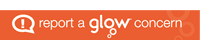Alves Primary School

Alves is ACEMaths homework – online games

The homework focus this term is on recall of multiplication and division facts.

1. multiplication facts for the 2,4, 5 and 10 times-tables, and corresponding division facts
2. multiplication facts to 12 x 12 and corresponding division facts
3. with increasing speed and confidence, multiplication facts to 12 x 12 and the corresponding division facts.

This is a selection of free games available online.

SUMS Mathematics – web